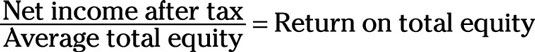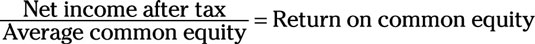##### Corporate Finance For Dummies, 2nd EditionImagine that you own equity in a company. You’d probably want to know how much value the company is making for you, the stockholder. The good news is you can calculate the amount of income a company is able to generate with the equity you have invested in it by using the return on total equity metric, which looks like this:To put this equation to work for you, follow these steps:

1. Find net income after tax near the bottom of the income statement.

2. Find the average total equity by using the balance sheets from the current year and the previous year: Add the total equity from the current year and the previous year, and then divide the sum by 2.

3. Divide the current year’s net income after tax by the average total equity to find the return on total equity.

Regardless of whether a company has only common shares or also has preferred shares, this ratio takes all equity into account. So if you’re a preferred shareholder, this is the return on equity you want to be concerned about.

Return on common shares determines how much income a company can generate based only on the value of its common stock, discounting all other forms of equity. If a company issues only common stock and no other types, this metric will produce exactly the same number as return on total equity. Here’s what return on common equity looks like:To use this metric, follow these steps:

1. Find net income after tax near the bottom of the income statement.

2. Find average common equity by using the balance sheets from the current year and the previous year: Add the common equities from the current year and the previous year, and then divide the sum by 2.

3. Divide the current year’s net income after tax by the average common equity to find the return on common equity.

Return on common shares is an important ratio for any stockholder to know because it shows how well a company is performing in regards to the interests of those who hold equity.